Next: Conclusions Up: A dynamical model for Previous: The dynamical model

# Results

The synthetic ECG (Fig. 5) illustrates the modulation of the QRS-complex due to RSA. Observational uncertainty is incorporated by adding normally distributed measurement errors with mean zero and standard deviation 0.025 mV (Fig. 6a), yielding a similar signal to a segment of real ECG from a normal human (Fig. 6b). In order to illustrate the dynamics of the RR-intervals obtained from this synthetic ECG, peak detection was used to identify the times of the R-peaks. In the noise-free case, a simple algorithm which looks for local maxima within a small window is sufficient. For ECGs with noise and artefacts it may be necessary to use more complicated methods [2,3]. A comparison between the continuous process with power spectrum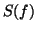given by (3) and the piecewise constant reconstruction of the RR-process obtained from the R-peak detection (Fig. 7) illustrates the measurement errors that arise when computing heart rate variability statistics from RR-intervals. The RR-intervals (Fig. 8a) and corresponding instantaneous heart rate (Fig. 8b) in units of beats per minute (bpm) for a mean of 60 bpm and standard deviation of 5 bpm display variability due to both RSA and Mayer waves. A spectral estimation technique for unevenly sampled time series, the Lomb periodogram [15,16], was used to calculate the power spectrum (Fig. 8c) from the RR tachogram, derived from 5 minutes of data as recommended by [7,10]. Despite the loss of information in going from the continuous process to the piecewise constant reconstruction, a comparison between Fig. 4 and Fig. 8c illustrates that it is still possible to obtain a reasonable estimate of the power spectrum.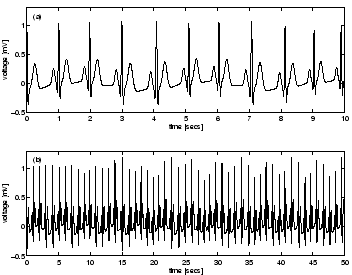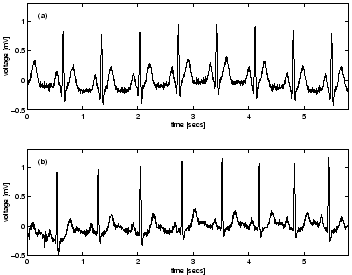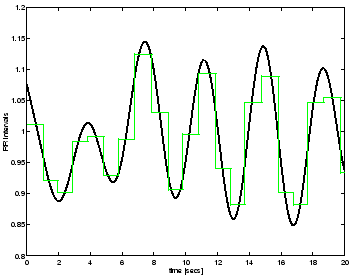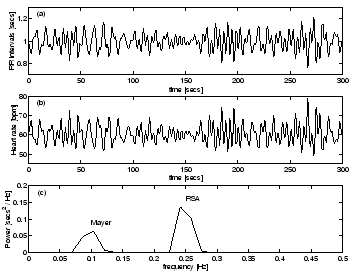An increase in the RR-interval implies that the trajectory has more time to get pushed into the peak and trough given by the R and S events. This is reflected by the strong correlation between the RR-intervals and the RS-amplitude as shown in Fig. 9. A technique for deriving a measure of the rate of respiration from the ECG has been proposed [5,6]. This ECG-derived respiratory signal (EDR) is of clinical use in situations where the ECG, but not respiration, is recorded. The synthetic ECG provides a means of testing the robustness of such techniques against noise and the effects of different sampling frequencies.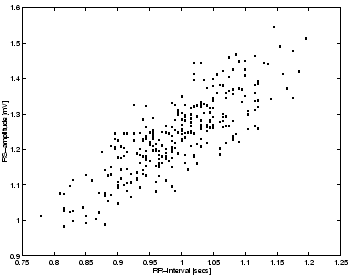As a consequence of constructing the model with a variable angular frequency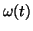, the time taken to move from the Q event to the T event, known as the QT-interval, varies with the RR-interval on a beat-to-beat basis. The relationship between the QT-interval and the RR-interval is linear as shown in Fig. 10. Such a linear relationship has been reported for real ECGs and has been used to calculate a corrected QT-interval . It is interesting that this relationship is a direct consequence of the model. Furthermore it may be possible to use the model to assess how much of the variation in the QT-interval is due to RR-interval variability so that this effect can be factored out.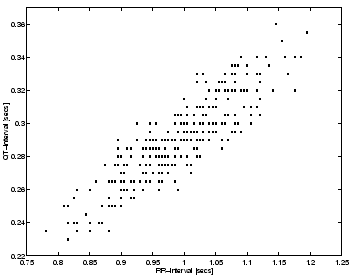Next: Conclusions Up: A dynamical model for Previous: The dynamical model
2003-10-08